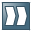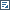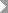Show TOCCreating Formula Expressions

In this step, we will build up a simple taxation model with three different tax calculation formulas for low, mid, and high incomes. The general approach is to divide an employee's gross salary into three segments and apply an individual tax rate to each of the segments (progressive taxation). The resulting tax amount for each segment will then be added to the total tax amount to be paid on the gross salary. The formula expressions will later be used in a decision tree.

Procedure

1. To create the formula expression, you have to navigate to the application in the Object Manager panel. To do so, choose the Back button. (You could as well choose the application from the Recently Used list.)

2. Under the Detail section, choose the Contained Objects tab.

The Contained Objects tab page opens.

3. Choose Expression from the Type field and choose Create Object.

4. In the Object Creation dialog that appears, choose Formula from the Type field.

5. Enter Calculate_tax_for_low_salary in the Name field.

6. Enter Amount to be paid in the Short Text field.

7. Choose Create and navigate to object.

You are navigated to the formula builder page.

8. Assign a result data object by choosing(Graphical Access).

9. In the context menu, choose Select....

10. In the Context Query dialog box that appears, select PAYABLE_TAX_AMOUNT. This is the data object that you have already created in the context of defining a result data object for the function.

It is added as the result data object.

11. In the context menu of the formula field, chooseInsert Data ObjectSelect.

12. In the Context Query dialog box that appears, select GROSS_SALARY. Again, this is a data object you have already created before in the context of defining the function's context data.

The data object gets added to the formula.

13. Choose the* button.

14. Choose the Number button.

15. In the Insert dialog box that appears, enter 0.1 and choose OK.

The number appears in the Formula field.

16. Save the formula expression.

17. Create the following formula expressions for the mid and high salary segments using the above procedure.

Name of the Formula Expression

Result Data Object

Formula

Calculate_Tax_for_med_salary

Payable_Tax_Amount

(25000 EUR * 0.1) + (Gross_Salary - 25000 EUR) * 0.2

Calculate_Tax_for_high_salary

Payable_Tax_Amount

(25000 EUR * 0.1) + (75000 EUR * 0.2) + (Gross_Salary - 100000 EUR * 0.3)Note

When you look at the fomulas in Expert Mode, amounts will be displayed, for example, as 25000\$EUR. Don't let yourself get confused by the “\$EUR” part. The salary currency is Euro (currency code EUR), while the \$ sign is inserted automatically by the system as an internal separator between the number and the currency part of the amount. This is needed for the formula to be properly parsed by the system. If the currency in the example was US Dollars, the formula editor would display this as \$USD in Expert Mode.

End of the note.